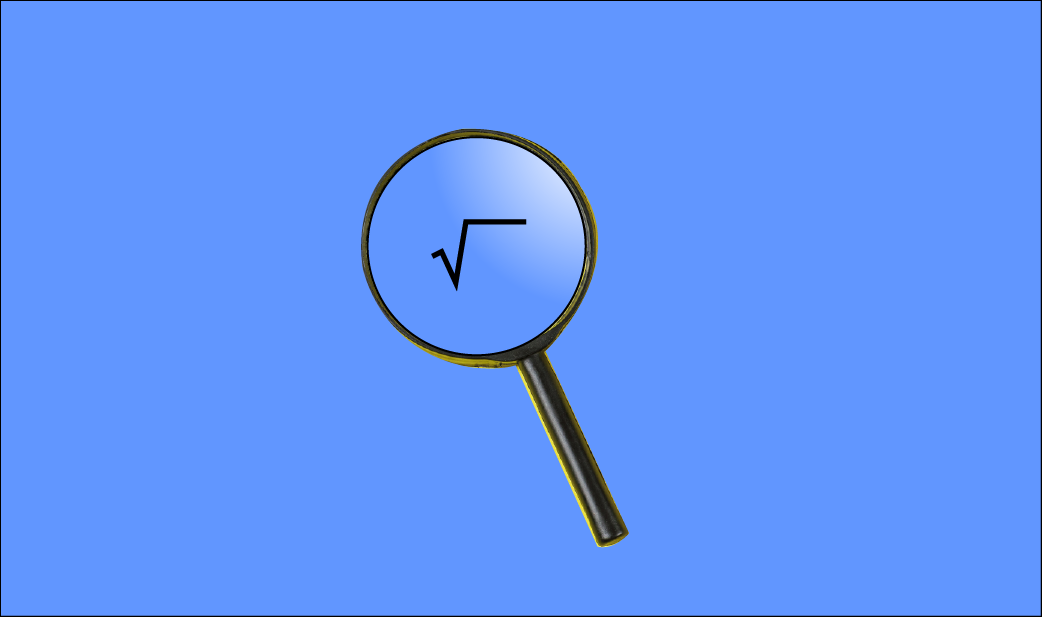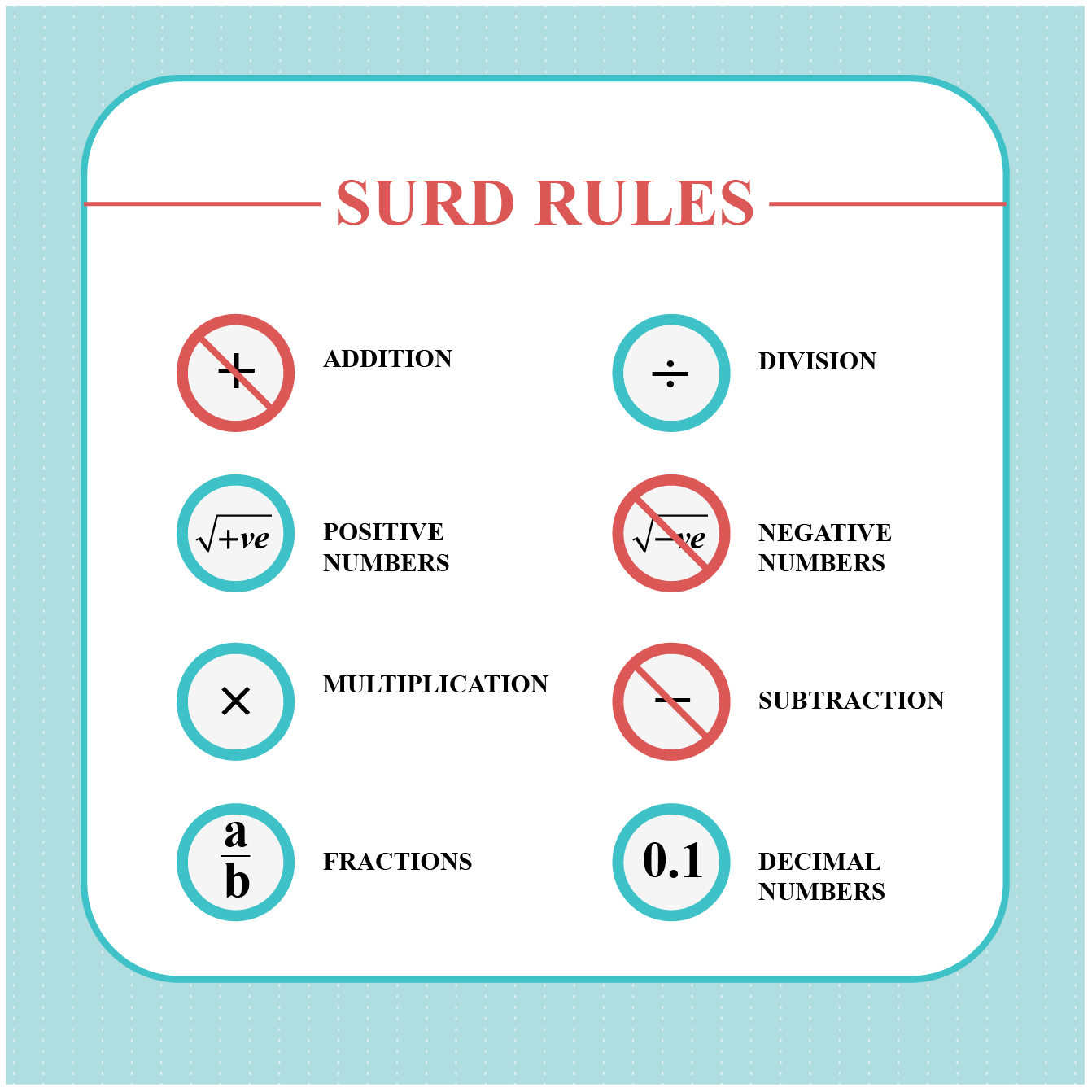# Surds

Surds

The Latin meaning of the word "Surd" is deaf or mute.  In earlier days, Arabian mathematicians called rational numbers and irrational numbers as audible and inaudible. Since surds form are made of irrational numbers, they were referred to as asamm (deaf, dumb) in Arabic language, and were later translated in Latin as surds.With this awareness, let's move ahead to learn the working of surds in math.

In this mini-lesson, we shall explore the topic of surds, by finding answers to questions like what do you mean by surds, what are the rules of surds, and simplifying calculations with surds.

## Lesson Plan

 1 What Do You Mean by Surds? 2 Think out of the Box! 3 Challenging Question 4 Solved Examples on Surds 5 Interactive Questions on Surds

## What Do You Mean by Surds?

Surd is simply used to refer to a number that does not have a root.

$$\sqrt 4$$, $$\sqrt 8$$, $$\sqrt 25$$ have roots as answers.

But $$\sqrt 6$$, $$\sqrt2$$, $$\sqrt20$$ do not have proper roots.

These number forms are termed as surds.

Also, these surds example expressed in exponential form would have fractions as powers.

\begin{align}\sqrt 6 &= 6^{\frac{1}{2}} \\ \sqrt2 &= 2^{\frac{1}{3}} \end{align}

According to surds definition, there are three different types of surds.

Pure Surds: A surd having only a single irrational number is called a pure surd. $\sqrt7,~ \sqrt11,~ \sqrt x^3$

Mixed Surds: A surd having a mix of a rational number and an irrational number is called a mixed surd. $x\sqrt y,~ 4\sqrt3,~ 8\sqrt5$

Compound Surds:  A surd composed of two surds is called a compound surd. $4 + \sqrt3,~ \sqrt5 + \sqrt2,,~\sqrt a + b\sqrt c$

## What Are the Rules of Surds?

A few rules of working with surds are:• Surds cannot be added.  $\sqrt a + \sqrt b \neq \sqrt(a + b)$
• Surds cannot be subtracted.$\sqrt a - \sqrt b \neq \sqrt(a - b)$
• Surds can be multiplied. $\sqrt a \times \sqrt b = \sqrt(a \times b)$
• Surds can be divided. $\frac{\sqrt a}{\sqrt b} = \sqrt\frac{a}{b}$
• Surds can be written in exponential form.   \begin{align} \sqrt a &= a^{\frac{1}{2}} \\ \sqrt[n] a&= a^{\frac{1}{n}}\end{align}Think Tank

Generally, surds cannot be added. But we can add similar surds. \begin{align}m\sqrt a + n\sqrt a &= (m +n)\sqrt a \\ 3\sqrt5 + 2\sqrt 5 &= 5\sqrt 5\end{align}

Now try to find the solution for the following surds. $2\sqrt5 + \sqrt3 + \sqrt 2 + \sqrt 5 + 2\sqrt3 = ?$

## Simplify Surd Calculation with Steps

Simplification of surds is needed for performing calculations.

There are two simple steps to surd simplification.

STEP - 1:  Split the number within the root into its prime factors.  $\sqrt50 = \sqrt(5 \times 5 \times 2)$

STEP-II:  Based on the root write the prime factors, outside the root. In case of square root, write one factor outside the root, for every two similar factors within the root.  $\sqrt(5 \times 5 \times 2) = 5\sqrt 2$

### Example

Let us use the above steps to perform the following calculation.

$\sqrt 18 + \sqrt50$

The above operation of addition is not possible without further simplification.

\begin{align}\sqrt18 + \sqrt50&= \sqrt (3 \times 3 \times 2) + \sqrt(5 \times 5 \times 2) \\ &= 3\sqrt2 + 5\sqrt2 \\ &=8\sqrt2\end{align}

## Solved Examples

 Example 1

Convert $$\sqrt 80$$ into mixed surd.

Solution

\begin{align}\sqrt 80 &=\sqrt (16 \times 5) \\ &= \sqrt(2 \times 2 \times 2 \times 2 \times 5 )\\ &= 2 \times 2 \times \sqrt 5 \\ &= 4\sqrt 5 \end{align}

 $$\therefore \sqrt 80 = 4 \sqrt 5$$
 Example 2

Find the product of $$4 \sqrt 3$$ and $$2 \sqrt 5$$

Solution

\begin{align} 4 \sqrt 3 \times 2 \sqrt 5 &= 4 \times 2 \times \sqrt3 \times \sqrt 5\\ &=8 \times \sqrt(3 \times 5) \\ &= 8\sqrt 15\end{align}

 $$\therefore \text {The product is }8\sqrt 15$$
 Example 3

Write the expansion of $$(4 + 3\sqrt 2)^2$$

Solution

\begin{align} (4 + 3\sqrt 2)^2 &= 4^2 + (3 \sqrt 2)^2 + 2.4.3\sqrt 2\\ &= 16 + 18 + 24\sqrt2 \\ &= 34 + 24\sqrt2\end{align}

 $$\therefore \text {The result of expansion is } 34 + 24\sqrt 2$$
 Example 4

Find the sum of  $$(2\sqrt 5 - 4\sqrt 2)$$ , $$(3\sqrt5 + 5 \sqrt 2)$$

Solution

\begin{align} (2\sqrt 5 - 4\sqrt 2) +(3\sqrt5 + 5 \sqrt 2) &=2\sqrt 5 - 4\sqrt 2 +3\sqrt 5 + 5 \sqrt 2 \\ &=5\sqrt 2– 4 \sqrt 2 +2\sqrt 5 +3\sqrt 5 \\ &=\sqrt 2 +5\sqrt 5 \end{align}

 $$\therefore \text {The sum is } \sqrt 2 +5\sqrt 5$$
 Example 5

Find the product of the surds $$(\sqrt 2 + 5\sqrt 3)$$ ,  $$(\sqrt 3 – 2\sqrt 5)$$

Solution

\begin{align} (\sqrt 2 + 5\sqrt 3) \times (\sqrt 3 – 2\sqrt 5)& =\sqrt 2(\sqrt 3 – 2\sqrt 5) +5\sqrt3(\sqrt 3 – 2\sqrt 5) \\ &=\sqrt 2 \times \sqrt 3 - \sqrt 2 \times 2\sqrt 5 +5\sqrt3 \times\sqrt 3 -5\sqrt3\times 2\sqrt 5 \\ &= \sqrt6 -2\sqrt10-5\sqrt9 -10\sqrt15 \\ &= -5\times3+\sqrt6 -2\sqrt10 -10\sqrt15 \\&=-15+\sqrt6 -2\sqrt10 -10\sqrt15 \end{align}

 $$\therefore \text{The product is} -15+\sqrt6 -2\sqrt10 -10\sqrt15$$Challenging Question

Find the square root  of $$\sqrt {5 + 2\sqrt6}$$

Hint: You may use the formula  $$(a + b)^2 = a^2 + b^2 + 2ab$$, and here we have $$a^2 + b^2 = 5; ab = 6$$

## Interactive Questions on Surds

Here are a few activities for you to practice. Select/Type your answer and click the "Check Answer" button to see the result.

## Let's Summarize

The mini-lesson targeted the fascinating concept of surds. The math journey around surds starts with what a student already knows, and goes on to creatively crafting a fresh concept in the young minds. Done in a way that not only it is relatable and easy to grasp, but also will stay with them forever. Here lies the magic with Cuemath.

At Cuemath, our team of math experts is dedicated to making learning fun for our favorite readers, the students!

Through an interactive and engaging learning-teaching-learning approach, the teachers explore all angles of a topic.

Be it worksheets, online classes, doubt sessions, or any other form of relation, it’s the logical thinking and smart learning approach that we, at Cuemath, believe in.

## FAQs on Surds

### 1. What are surds in math?

Surds definition in math refers to the numbers that do not have answers to their roots. A few examples of surds as $$\sqrt5,~ \sqrt7,~ 2 +\sqrt3,~ \sqrt6 + 2\sqrt3$$.

### 2. How to rationalize surds?

To rationalize a surd we need to multiply the surd with its conjugate surd. To rationalize $$\sqrt5$$ we need to multiply it with $$\sqrt5$$.  $\sqrt5 \times \sqrt5 = 5$

### 3. How to solve surds?

To solve an expression in surds form we need to take prime factors of the number within the surd. Further, it is simplified by taking the possible prime factors outside the root symbol. $\sqrt18 = \sqrt(3 \times 3 \times 2) = 3\sqrt2$

### 4. What is a surd example?

Some of the surds examples are $$\sqrt11,~ 5\sqrt3,~ 17 + \sqrt3,~ \sqrt5 + \sqrt10$$

### 5. Can surds have negative numbers?

A surds form does not have negative numbers. Surds can only have positive numbers, decimals, and fractions.

### 6. Can a surd be a fraction?

A surds form can be a fraction also. A few examples of surd fractions are as follows. $\sqrt{\frac{3}{2}}, \frac{1}{\sqrt5}$

### 7. What are the types of surds?

There are three types of surds. Simple surds, mixed surds, and compound surds.

Simple surd is of the form $$\sqrt x$$. Some of the examples are $$\sqrt 5, ~\sqrt 0.8 ,~ \sqrt 17$$

Mixed surd are of the form $$x\sqrt y$$. A few examples are $$5\sqrt3,~2\sqrt7,~ 3\sqrt2$$

Compound surd is a sum of two or more surds. Examples include $$5 + \sqrt2,~4\sqrt3 + 3\sqrt5,~ 5 + \sqrt3 + \sqrt11$$

### 8.  What are conjugate surds?

Conjugate surds are the surds which, on multiplying with the given surd, convert it into a non-surd.

Conjugate surd of $$\sqrt5$$ is $$\sqrt 5$$

Conjugate surd of $$(2 + \sqrt 3 )$$ is $$(2 - \sqrt 3)$$

Numbers and Number Systems